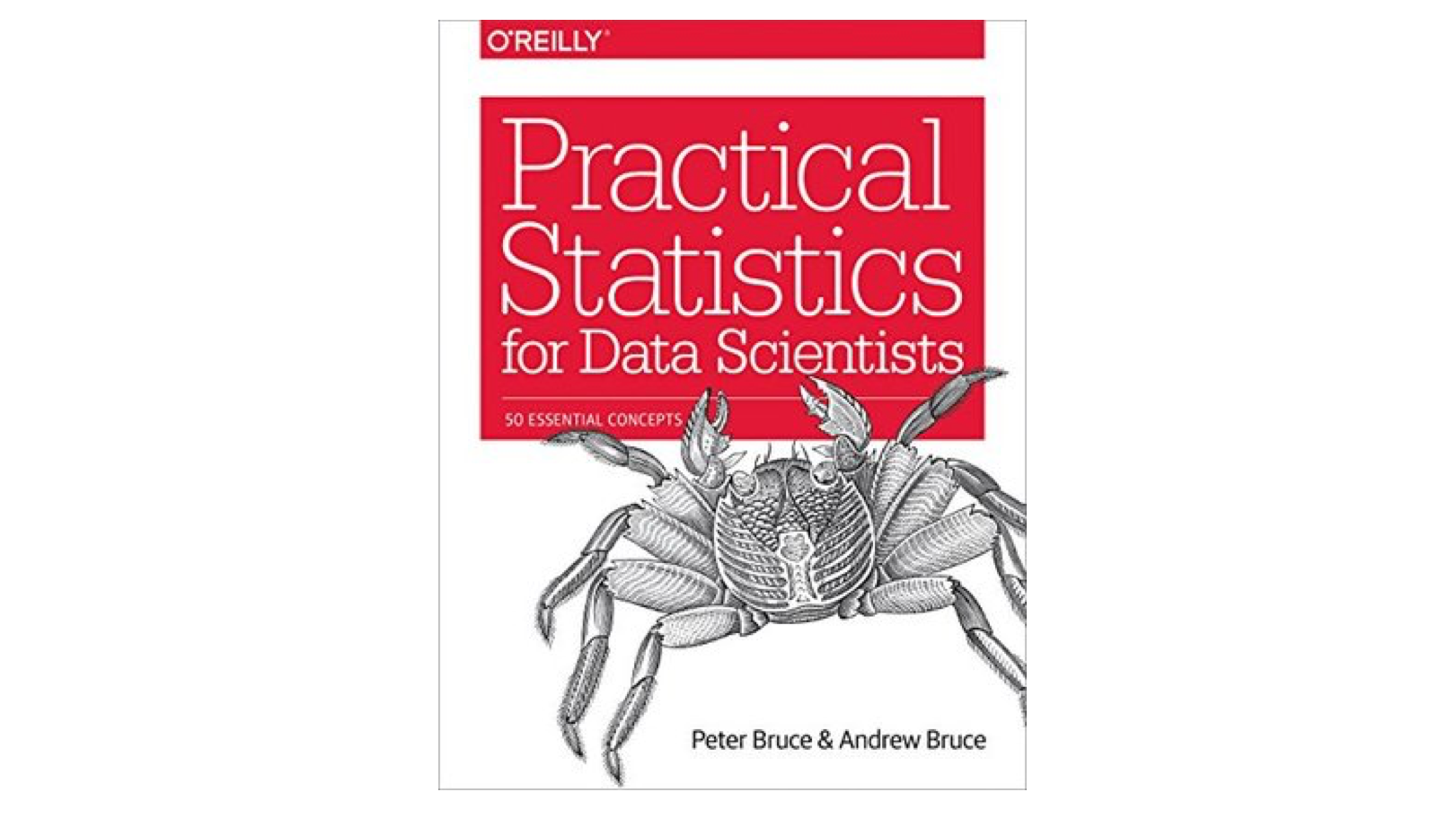## Practical Statistics for Data Scientists

March 26 2020A second edition, *Practical Statistics for Data Scientists: 50+ Essential Concepts Using R and Python* is scheduled for publication on June 9, 2020.

## Who is this book for?

According to the preface:

"This book is aimed at the data scientist with some familiarity with the R programming language, and with some prior (perhaps spotty or ephemeral) exposure to statistics."
"Two goals underlie this book:
1) to lay out, in digestible, navigable, and easily referenced form, key concepts from statistics that are relevant to data science, and
2) to explain which concepts are important and useful from a data science perspective, which are less so, and why."

## Book outline

1. Exploratory Data Analysis Elements of Structured Data
Rectangular Data
Estimates of Location
Estimates of Variability
Exploring the Data Distribution
Exploring Binary and Categorical Data
Correlation
Exploring Two or More Variables
Summary
2. Data and Sampling Distributions Random Sampling and Sample Bias
Selection Bias
Sampling Distribution of a Statistic
The Bootstrap
Confidence Intervals
Normal Distribution
Long-Tailed Distributions
Student’s t-Distribution
Binomial Distribution
Poisson and Related Distributions
Summary
3. Statistical Experiments and Significance Testing A/B Testing [March 23, 2020]
Hypothesis Tests [March 25, 2020]
Resampling [March 26, 2020]
Statistical Significance and P-values
t-Tests
Multiple Testing
Degrees of Freedom
ANOVA
Chi-Square Test
Multi-Arm Bandit Algorithm
Power and Sample Size
Summary
4. Regression and Prediction Simple Linear Regression
Multiple Linear Regression
Prediction Using Regression
Factor Variables in Regression
Interpreting the Regression Equation
Testing the Assumptions: Regression Diagnostics
Polynomial and Spline Regression
Summary
5. Classification Naive Bayes
Discriminant Analysis
Logistic Regression
Evaluating Classification Models
Strategies for Imbalanced Data
Summary
6. Statistical Machine Learning K-Nearest Neighbours
Tree Models
Bagging and the Random Forest
Boosting
Summary
7. Unsupervised Learning Principal Components Analysis
K-Means Clustering
Hierarchical Clustering
Model-Based Clustering
Scaling and Categorical Variables
Summary

## Impressions

### Strengths

Book is divided up into fairly bite-sized concepts, which provide useful chunks to guide learning

### Weaknesses

The amount of equations included in the text can interrupt flow and be confusing to readers without a significant statistics background